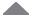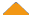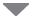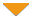Run Code  | API  | Code Wall  | Misc  | Feedback  | Login  | Theme  | Privacy  | Patreon

## Easter Holiday Calculator

 run  | edit  | history  | help2``````/// /// Returns the date of Easter for the given year. /// /// The year to compute the date of Easter for. /// A with the date of Easter for the given year. /// /// Computus is Latin for 'computation' and determines the calendar date of Easter. This calculation is called /// the "Meeus/Jones/Butcher" algorithm. /// private static DateTime Computus (int year) { // This algorithm was found at http://en.wikipedia.org/wiki/Computus int a = year % 19; int b = year / 100; int c = year % 100; int d = b / 4; int e = b % 4; int f = (b + 8) / 25; int g = (b - f + 1) / 3; int h = ((19 * a) + b - d - g + 15) % 30; int i = c / 4; int k = c % 4; int l = (32 + (2 * e) + (2 * i) - h - k) % 7; int m = (a + (11 * h) + (22 * l)) / 451; int n = h + l - (7 * m) + 114; int month = n / 31; int day = (n % 31) + 1; return new DateTime(year, month, day); } ``````   by  Jesse C. Slicer, 3 years ago Please log in to post a comment.